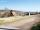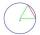Diagonal

Can be a diagonal of diamond twice longer than it side?

Result

Solution:Leave us a comment of example and its solution (i.e. if it is still somewhat unclear...):Be the first to comment!Next similar examples:

1. DiagonalCan a rhombus have the same length diagonal and side?
2. Rhombus 29One of the diagonals of a rhombus is equal to a side of the rhombus. Find the angles of the rhombus.
3. AcreagePlot has a diamond shape, its side is 25.6 m long and the distance of the opposite sides is 22.2 meters. Calculate its acreage.
4. ChordPoint on the circle is the end point of diameter and end point of chord length of radius. What angle between chord and diameter?
5. MidpointsTriangle whose sides are midpoints of sides of triangle ABC has a perimeter 45. How long is perimeter of triangle ABC?
6. 3-bracket 3Two angles in a triangle are 90° and 60°. Has triangle at least two equal sides?
7. 4-gonIt is true that a 4-gon whose two sides are parallel and the other two has equal length, is a parallelogram?
8. Center traverseIt is true that the middle traverse bisects the triangle?
9. Triangle ABCConstruct a triangle ABC is is given c = 60mm hc = 40 mm and b = 48 mm analysis procedure steps construction
10. Rhombus anglesIf one angle in the rhombus is 159°, what is it neighboring angle in rhombus?
11. Simple equationSolve for x: 3(x + 2) = x - 18
12. Obtuse angleWhich obtuse angle is creating clocks at 17:00?
13. BicycleThe bicycle pedal gear has 36 teeth, the rear gear wheel has 10 teeth. How many times turns rear wheel, when pedal wheel turns 120x?
14. Seeds 2How many seeds germinated from 1000 pcs, when 23% no emergence?
15. IronIron ore contains 57% iron. How much ore is needed to produce 20 tons of iron?
16. AverageThe arithmetic mean of the two numbers is 71.7. One number is 5. Calculate the second number.
17. ClassIn 7.C clss are 10 girls and 20 boys. Yesterday was missing 20% of girls and 50% boys. What percentage of students missing?